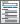Home  /  Products  /  Features  /  Multilevel models

## Multilevel mixed-effects models

Whether the groupings in your data arise in a nested fashion (students nested in schools and schools nested in districts) or in a nonnested fashion (regions crossed with occupations), you can fit a multilevel model to account for the lack of independence within these groups. Fit models for continuous, binary, count, ordinal, and survival outcomes. Estimate variances of random intercepts and random coefficients. Compute intraclass correlations. Predict random effects. Estimate relationships that are population averaged over the random effects. And much more.

Outcomes and regression estimators

Types of models

• Two-, three-, and higher-level models
• Nested (hierarchical) models
• Crossed models
• Mixed models
• Balanced and unbalanced designs

Types of effects

• Random intercepts
• Random coefficients (slopes)
• Variances of random effects (variance components)
• Fixed effects (regression coefficients)

Effect covariance structures• Identity—shared variance parameter for specified effects with no covariances
• Independent—unique variance parameter for each specified effect with no covariances
• Exchangeable—shared variance parameter and single shared covariance parameter for specified effects
• Unstructured—unique variance parameter for each specified effect and unique covariance parameter for each pair of effects
• Compound—any combination of the above

Error (residual) structures for linear models

• Independent
• Exchangeable
• Autoregressive
• Moving average
• Exponential
• Banded
• Toeplitz
• Flexible
• Unstructured

Estimation methods• Maximum likelihood (ML)
• Restricted maximum likelihood (REML)
• Laplacian approximation
• EM method starting values
• Kenward–Roger
• Satterthwaite
• ANOVA
• Repeated-measures ANOVA
• Residual

Constraints

• Linear constraints on fixed parameters
• Linear constraints on variance components
• Sampling weights
• Weights at each level of model
• Cluster–robust SEs allowing for correlated data

Multiple imputation• View and run all postestimation features for your command
• Automatically updated as estimation commands are run

Estimates of random effects

Predictions

Other postestimation analysis

• Automatically create indicators based on categorical variables
• Form interactions among discrete and continuous variables
• Include polynomial terms
• Perform contrasts of categories/levels
Watch Introduction to Factor Variables in Stata tutorials